# Smart Antenna and Beamforming, Understanding with GNU : Part 2

1. There are four types of antenna field regions. Out of those four regions, we discussed about radiation pattern of far field region of an antenna. Also, we plotted a 3D radiation pattern of an antenna with a given radiation intensity.
2. If we have single antenna then It would require a very tall antenna to direct the radiation towards a specific user. This is the simplest scheme of directing a beam towards a specific user but because of obvious reasons, this scheme is impractical.
3. Antenna array has two or more number of antenna elements or antennas. So, the output of an antenna array is equal to the weighted sum of outputs from its elements.  And, if we take multiple antenna elements, arrange them in a specific way then by introducing time delay (we assumed that time-delay is similar to phase-shift) among signal that is coming out of each antenna element would result in a change in direction of the radiation or beam.  This scheme is known as phased array antenna system.
4. If we increase the number of antenna elements in an array, the output beam from antenna becomes narrower. And, introducing time delay changes the direction of the output beam.
5. If you noticed octave scripts in part-1 of this article, I used phase shift instead of time delay. We can easily convert time delay experienced by signal into a phase shift at a given frequency. Consider a linear antenna array system as depicted in Figure 1.  If any signal arrives at degree off at antenna boresight (the axis of maximum gain of a directional antenna.). Then, time delay can be given by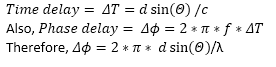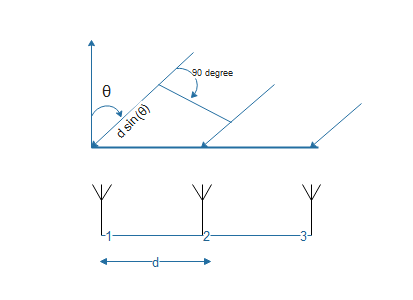Figure 1: Linear antenna array with three antenna elements, signal arriving at angle θ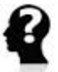Question to Think About:

• What will happen if we vary the distance between antenna array elements? How will antenna radiation pattern be impacted?
• What will happen if Antenna elements are not arranged in a linear fashion? How about a circular geometry

Smart Antenna System

Let’s define smart antenna system first in our words.

“Smart Antenna system consists of two major blocks. Firstly, an adaptive antenna array block and secondly, a sophisticated signal processing block. These two blocks improve the communication between transmitter and receiver by continuously adapting feedback to antenna array block. Consequently, radiation pattern (Beam) is optimized dynamically according to communication channel characteristics.”

Here, optimized beam pattern means optimizing its direction, shape, and range according to the communication channel.Figure 2: Simplest possible Smart Antenna system, w1, w2, w3, w4 are beamforming weights

Direction of Arrival or Angle of Arrival Estimation Techniques

As it can be seen from figure 2, smart antenna systems use a specific signal property called “Direction of Arrival”, here on referred as DOA, to calculate and update beamforming weights (w1, w2, w3, w4 and so on). So, the main goal here is to find or at least estimate DOA.

It is worth mentioning here that our aim is to calculate beamforming weights and there are many methods other than DOA estimation by which beamforming weights can be calculated. However, we will only focus here on DOA estimation and its use in beamforming weights calculation. Also, we will limit our discussion to uniform linear array (ULA) system, something that is represented in figure-1.

Now, it is to further note that there are many ways by which DOA can be estimated. Below given are some common DOA estimation techniques.

1. Root- MuSIC – Root Multi SIgnal Classification
2. ESPRIT – Estimation of Signal Parameters via Rotational Invariance
3. IQML – Ative quadratic maximum likelihood
4. Root-WSF – Weighted subspace fitting

All the above techniques can be characterized based on coherency, consistency and spectral efficiency.  As shown in below table:

 Method Consistency Coherent signals Statistical Performance Computations Root-MuSIC Yes Yes Good Eigenvalue Decomposition, Polynomial ESPRIT Yes Yes Good Eigenvalue Decomposition IQML Yes Yes Good Iterative Root-WSF Yes Yes Efficient Eigenvalue Decomposition, Least Square

In this article, we will focus on Root-MuSIC method of DOA estimation. But before discussing details of this method, It is important to review mathematics behind these methods in general.

Mathematics of Direction of Arrival or Angle of Arrival Estimation

• Eigenvalues and Eigen Vectors

Many DOA estimation algorithms work on the properties of eigenvalue decomposition. So, this concept is very important to understand.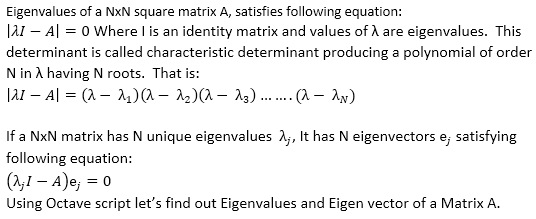Figure 3: eig() function for eigen analysis

In this example, the first column of e_vector is first eigen vector and its corresponding eigen value is 33.865. Similarly, the second column of e_vector is second eigen vector and its corresponding eigenvalue is -17.865

I would highly recommend lecture by Professor Gilbert Strang from MIT to understand the concept of Eigenvalues and Eigenvectors below.

• Array Correlation Matrix

Suppose there is an M-element antenna array and D-signals are arriving at it from Θ directions. As shown in the figure below:Figure 4: M-element antenna array with D-arriving SignalsAssuming that all the signals are zero mean, stationary processes then MxM Correlation matrix is composed of signal and noise samples at time k. And, it can be defined as follows: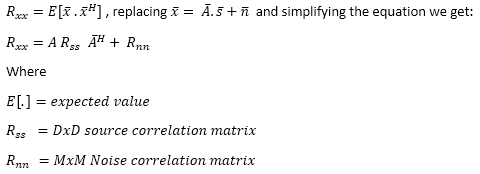Correlation matrix has M eigen values and associated M eigen vectors.  The largest eigenvalues correspond to the signals, while the rest are associated with the noise. So, this correlation matrix can be divided into two subspaces: signal subspace and noise sub-space. Mx(M-D) noise subspace and MxD signal subspace.

In that case (M-D) eigen vectors are corresponding to noise and D eigen vectors are corresponding to signal.  In other words, D uncorrelated signals incident on an M element array (M > D) produces D signal eigenvalues and M – D noise eigenvalues.

The signal eigenvectors associated with the correlation matrix are the array weights that have beams in the directions of the signals. The noise eigenvectors associated with the correlation matrix are the array weights that have nulls in the directions of the signals.

It is to note that if signals are not zero mean, stationary processes then correlation matrix is also known as a covariance matrix. So, the heart of the DOA estimation is eigen analysis of covariance matrix. Let’s understand eigen analysis of Covariance matrix further by using Octave.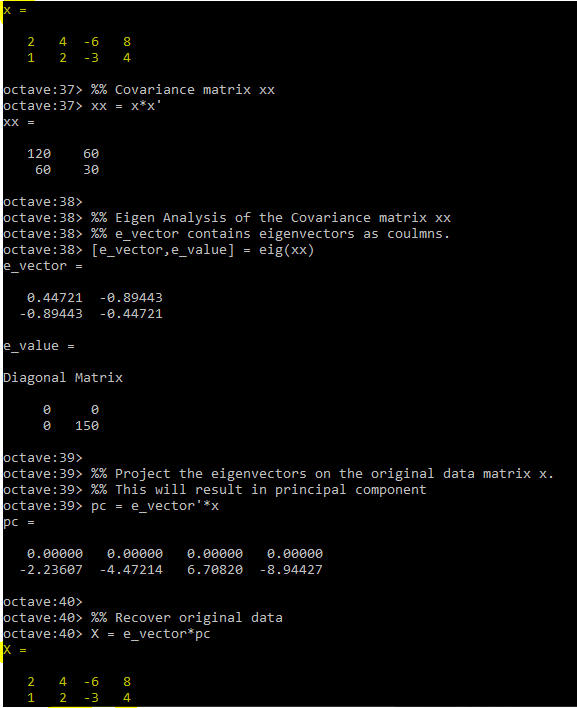It can be seen above that by doing eigen analysis of Covariance matrix we are able to recover original data. This is very useful and main building block of DOA estimation algorithms.

Now we have the basic understanding of smart antenna system. Part-3 of this Article will discuss details about Root-Music Algorithm for estimating DOA.

Article Submitted By Mr. Dheeraj Sharma